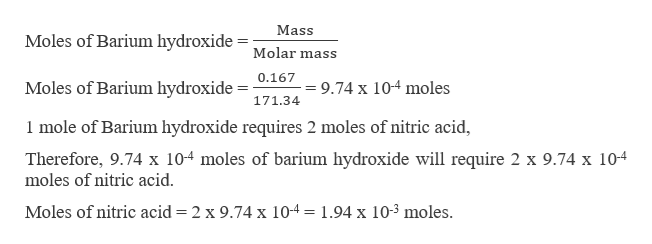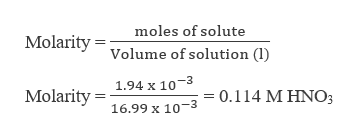What is the molarity of a solution of nitric acid if 0.167 g of barium hydroxide is required to neutralize 16.99 mL of nitric acid?

Question

What is the molarity of a solution of nitric acid if 0.167 g of barium hydroxide is required to neutralize 16.99 mL of nitric acid?

Step 1

The balanced chemical equation between barium hydroxide and nitric acid is shown as,

Step 2

To calculate the molarity, it is required to calculate the moles of nitric acid, which can be calculated as,help_outlineImage TranscriptioncloseMass Moles of Barium hydroxide Molar mass Moles of Barium hydroxide = 9.74 x 10-4 moles 171.34 1 mole of Barium hydroxide requires 2 moles of nitric acid Therefore, 9.74 x 104 moles of barium hydroxide will require 2 x 9.74 x 104 moles of nitric acid Moles of nitric acid = 2 x 9.74 x 10-4 1.94 x 103 moles. fullscreen
Step 3

Now, calculating the molarit...help_outlineImage Transcriptionclosemoles of solute MolarityVolume of solution (1) 1.94 x 10-3 Molarity 16.99 x 10-3 0.114 M HNO3 fullscreen

Want to see the full answer?

See Solution

Want to see this answer and more?

Our solutions are written by experts, many with advanced degrees, and available 24/7

See Solution
Tagged in

General Chemistry